# RS Aggarwal Solutions for Class 10 Maths Chapter 3 Linear Equations in Two Variables Exercise 3C

R S Aggarwal Solutions for Class 10 Maths Chapter 3 Linear Equations in Two Variables, contains solutions for exercise 3C questions. All questions are solved by experts at BYJU’S based on CBSE latest syllabus using step by step method. R S Aggarwal solutions on exercise 3c helps student’s solving simultaneous linear equations using cross multiplication method. Download RS Aggarwal Solutions of Class 10  maths and clear all your doubts related to linear equations.

## Download PDF of RS Aggarwal Solutions for Class 10 Maths Chapter 3 Linear Equations in Two Variables Exercise 3C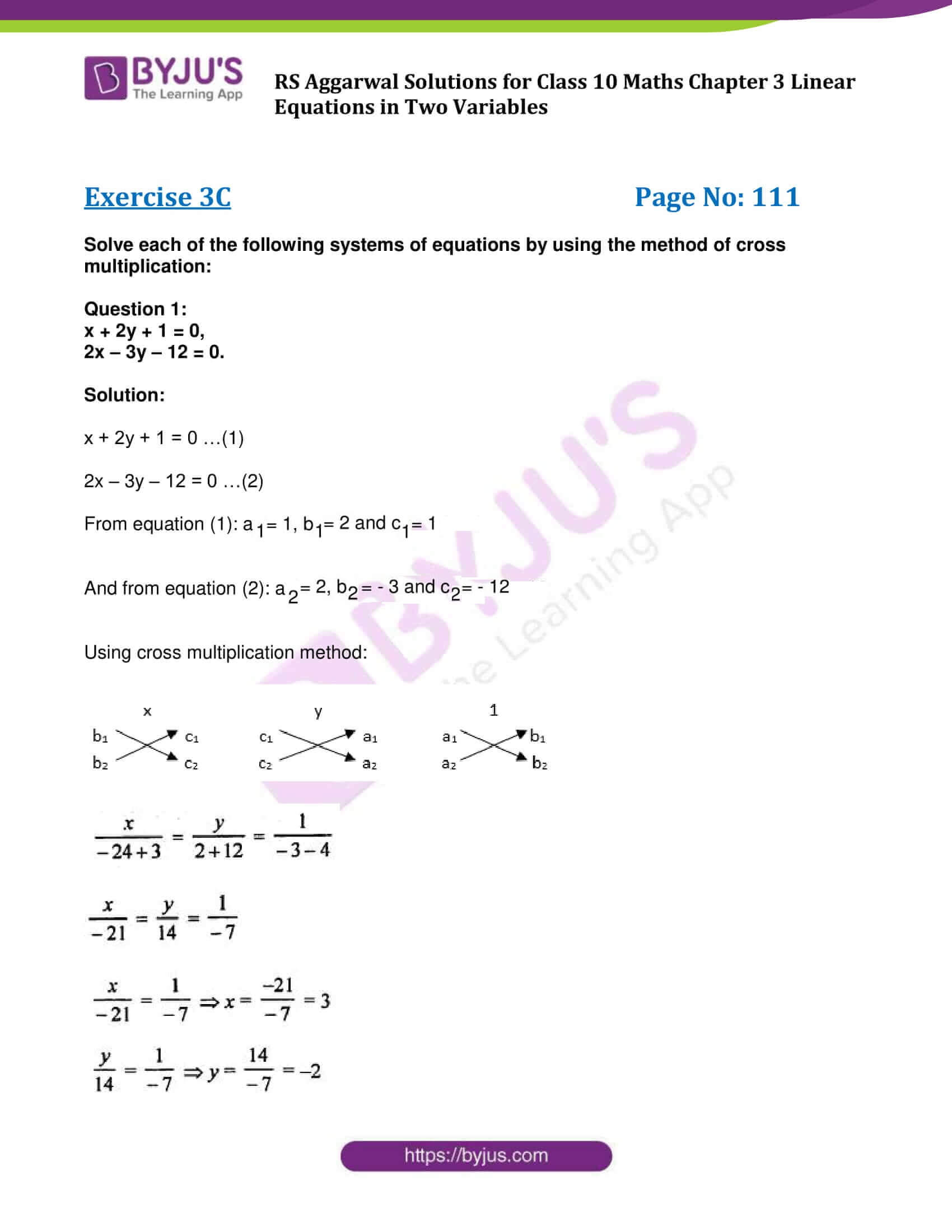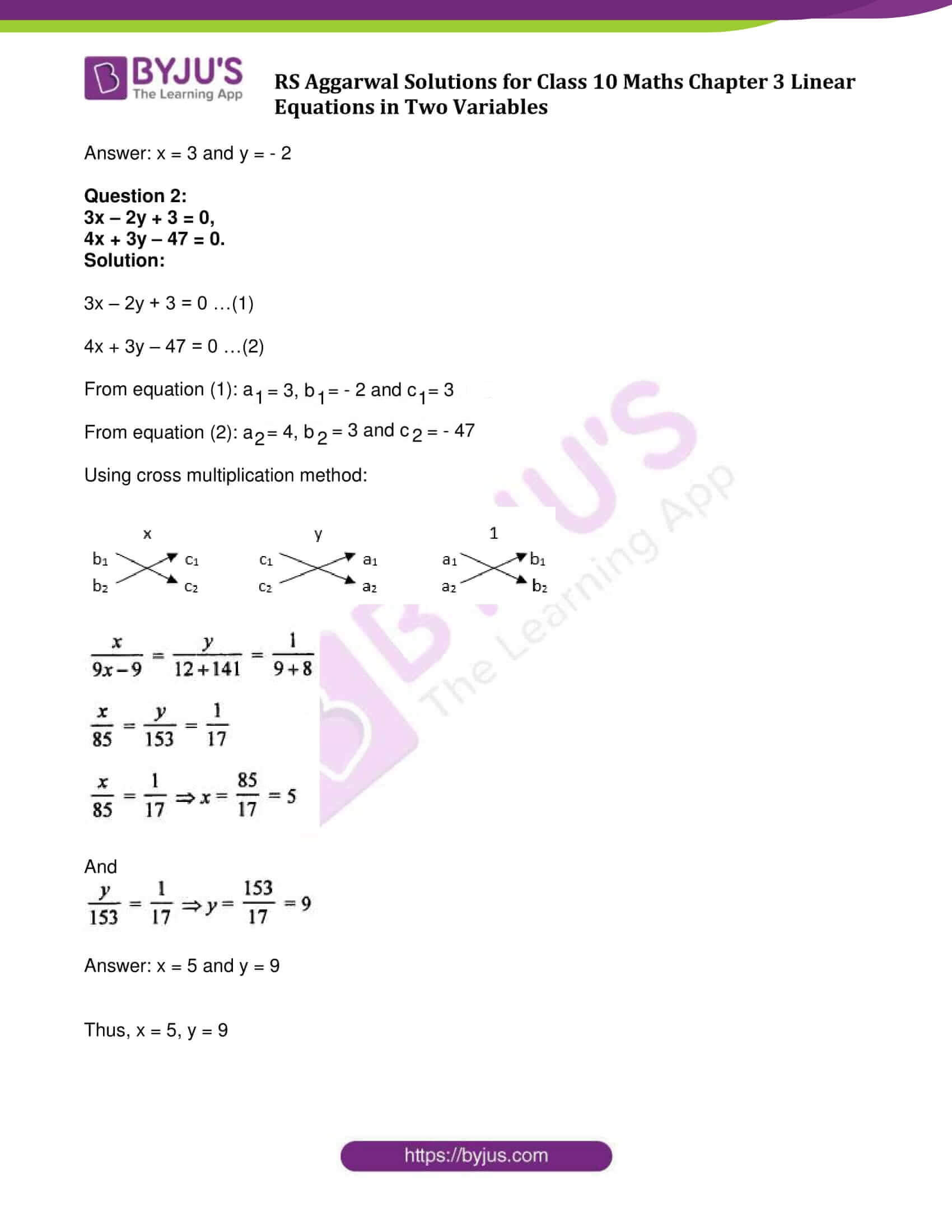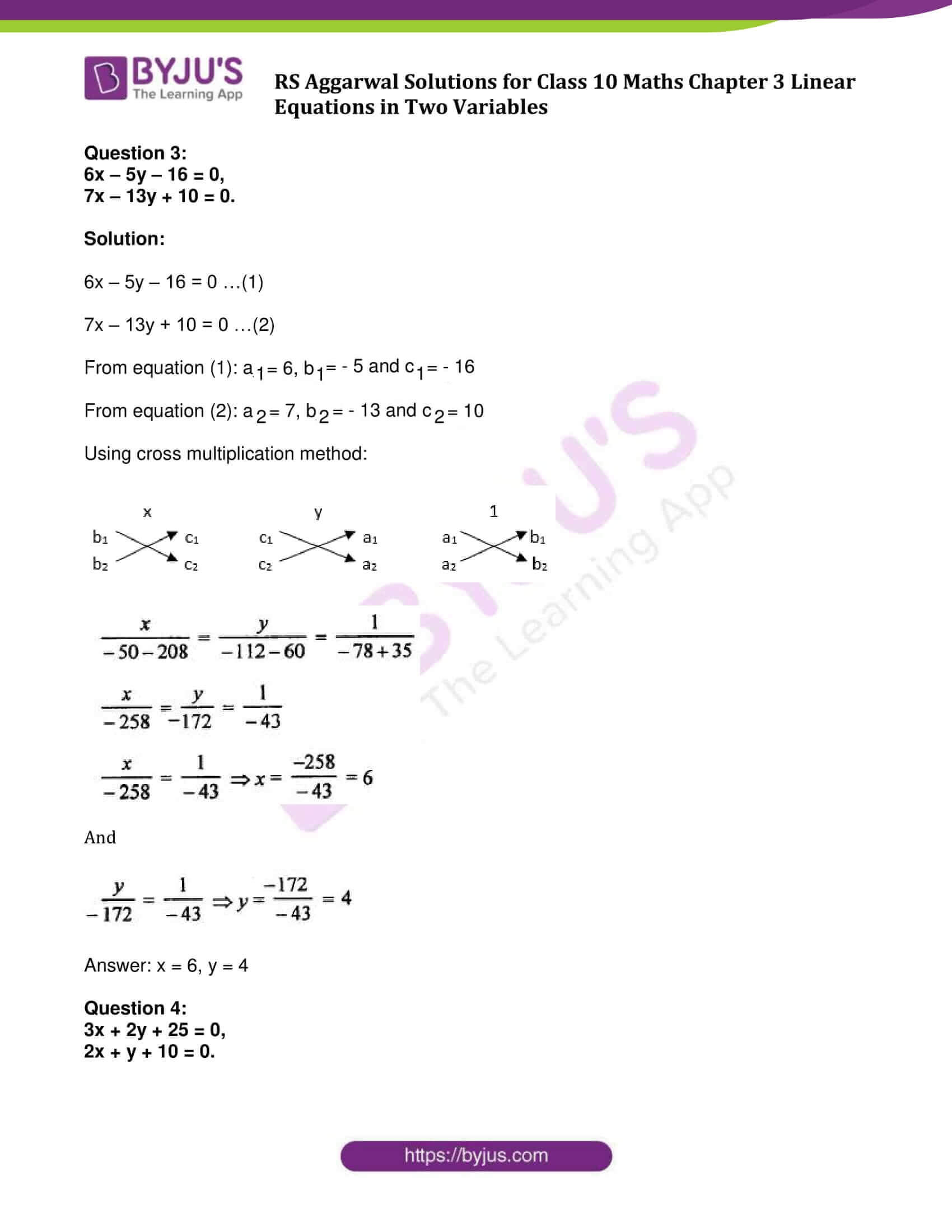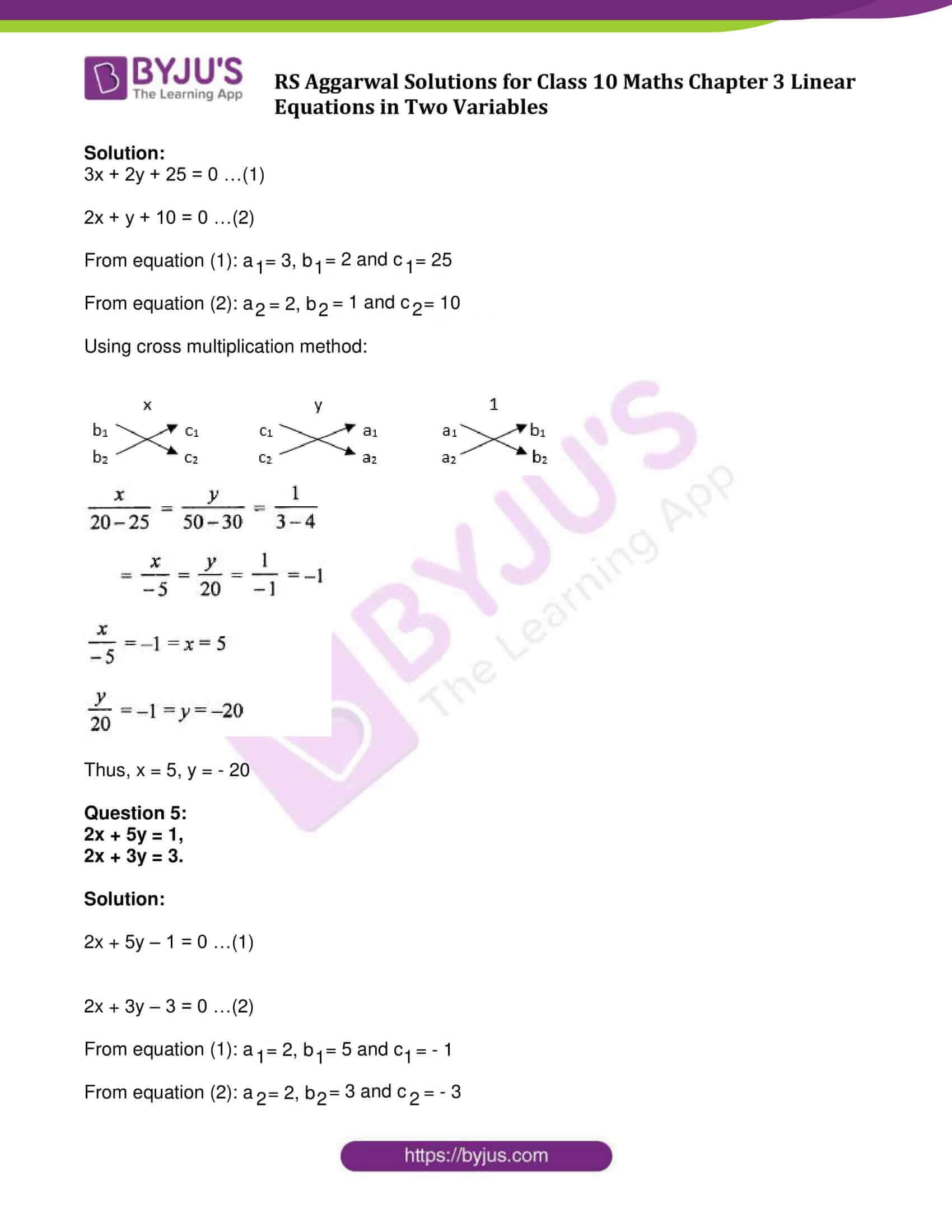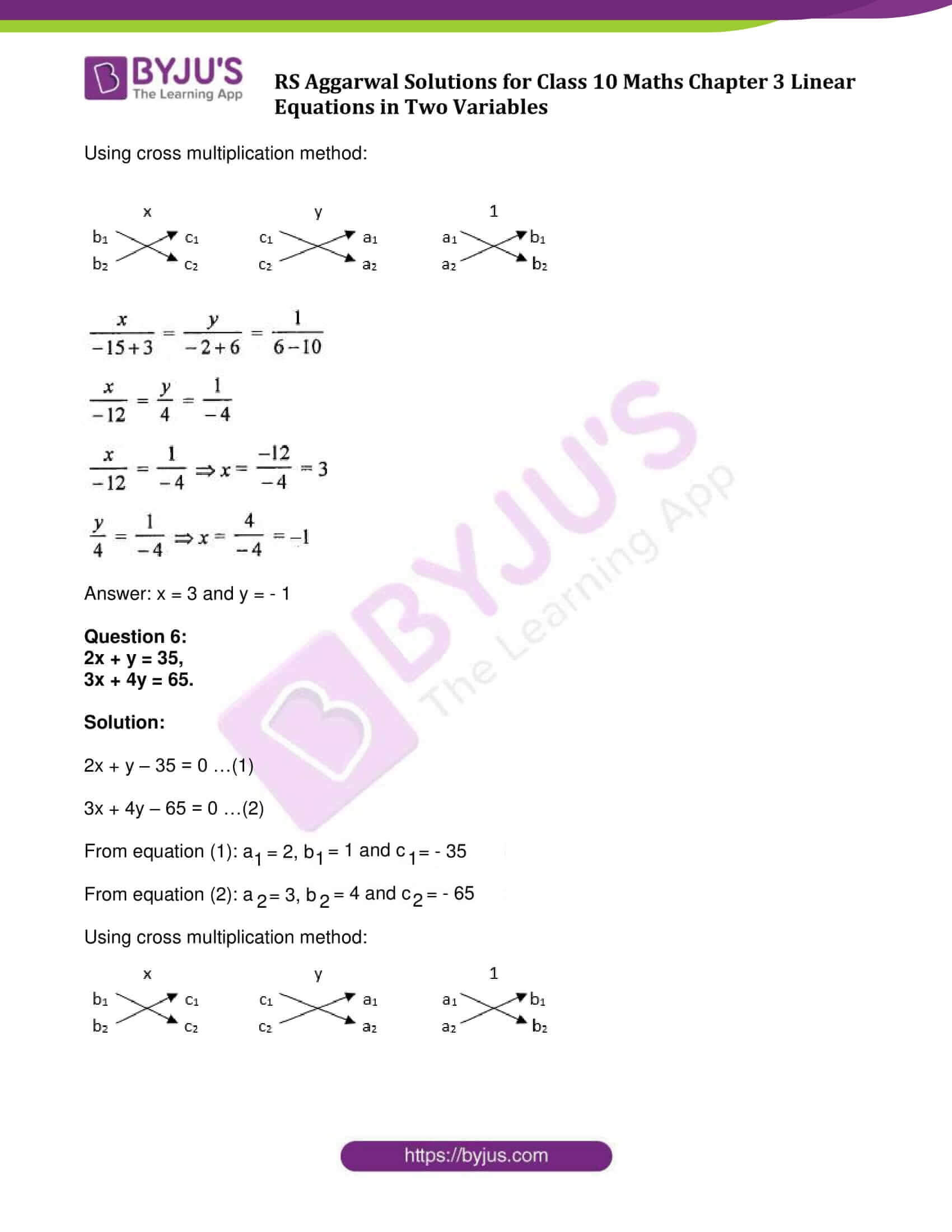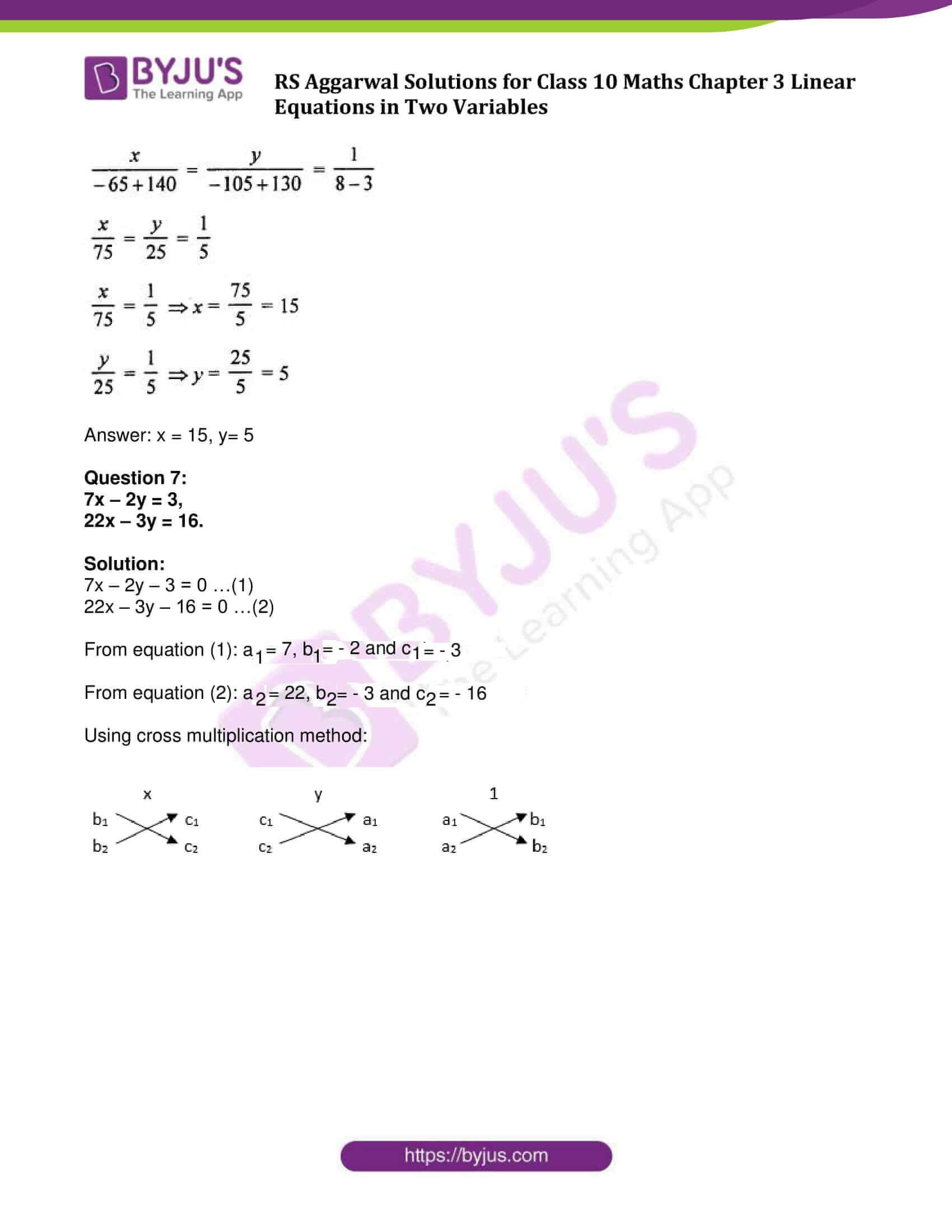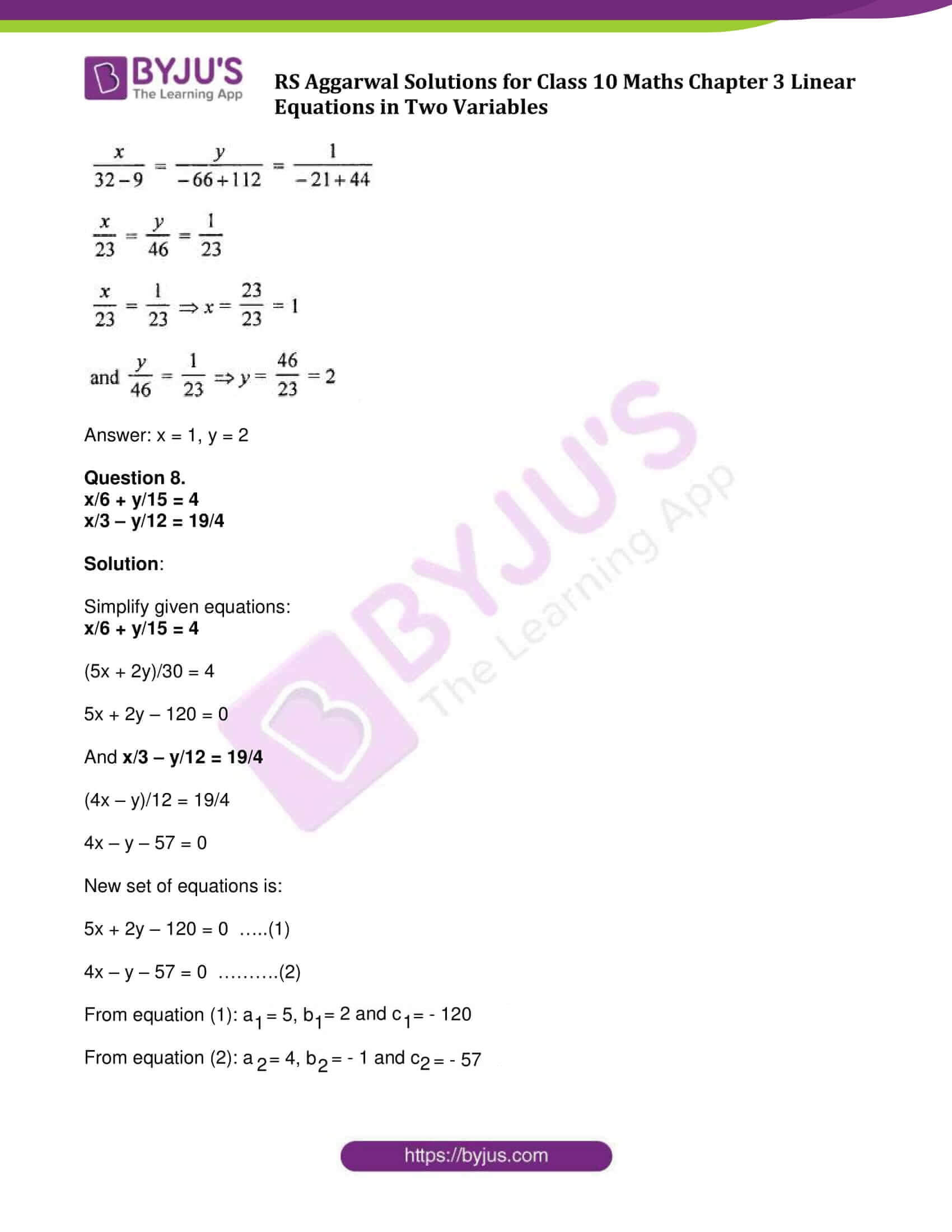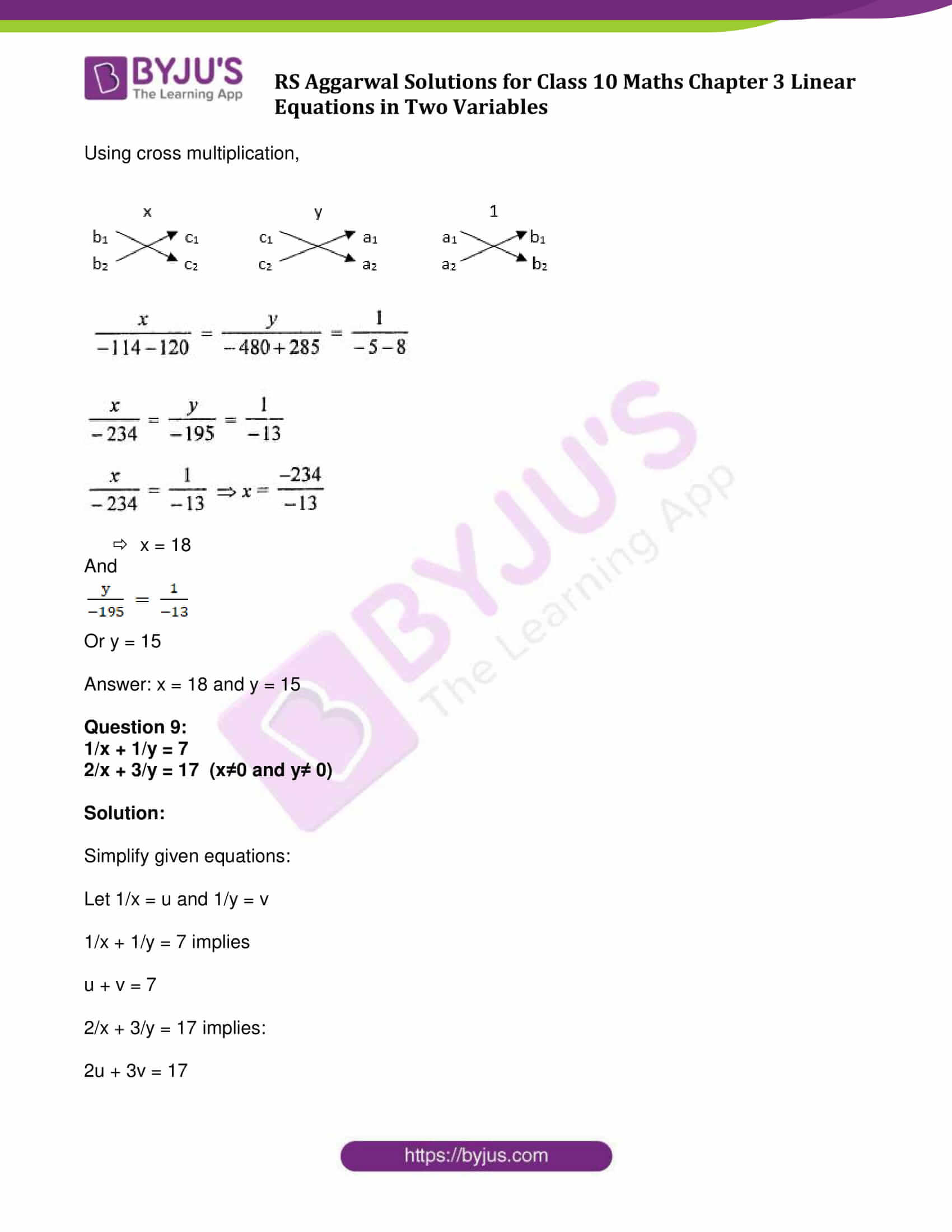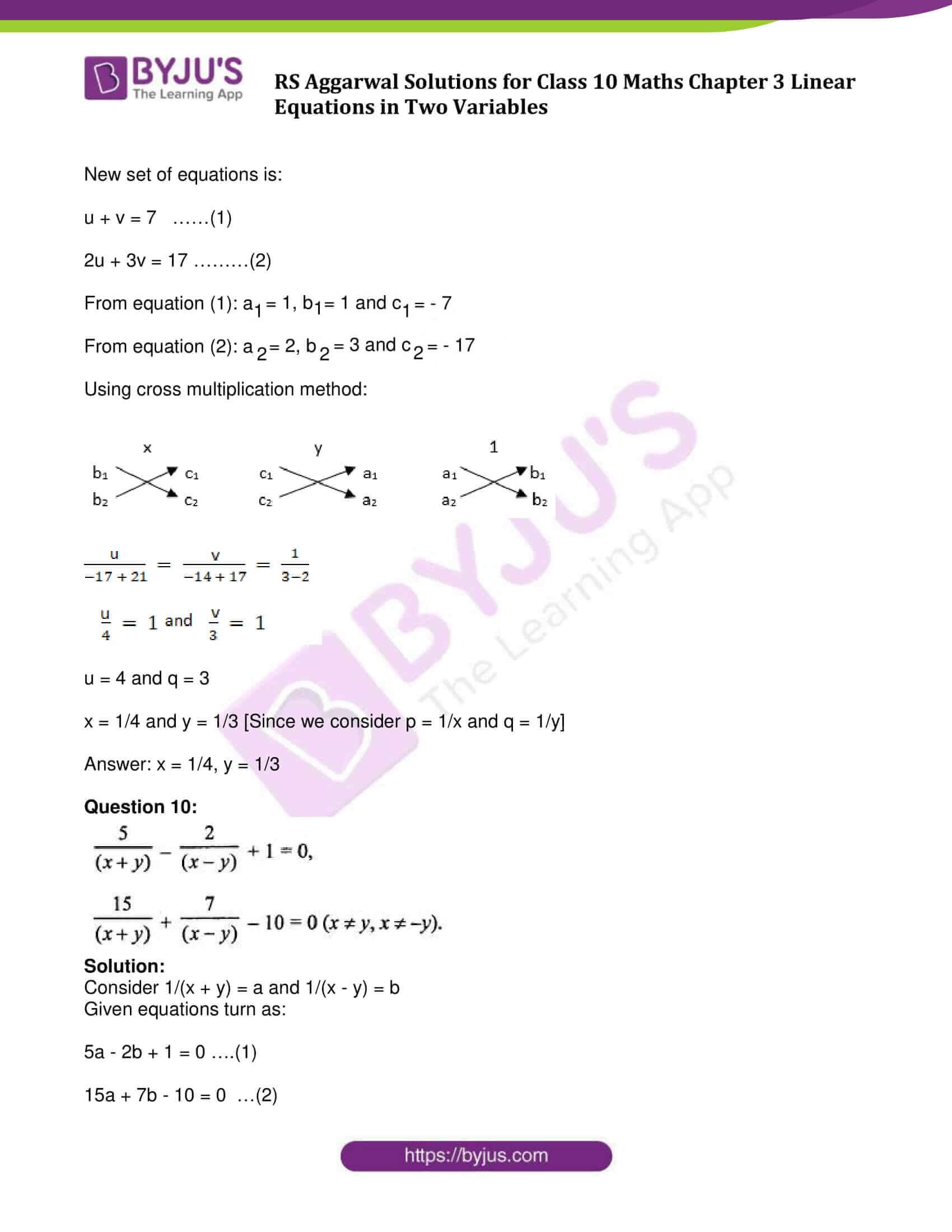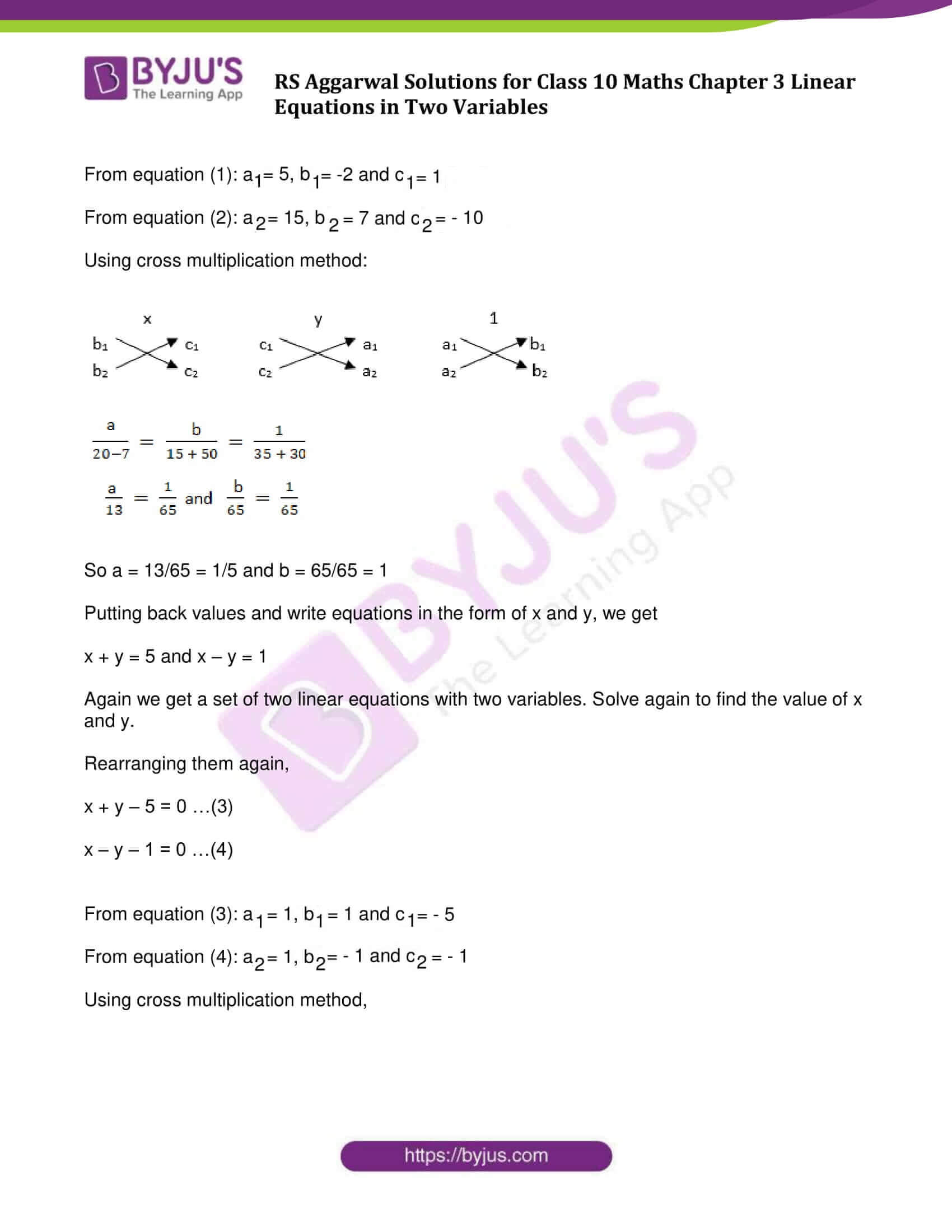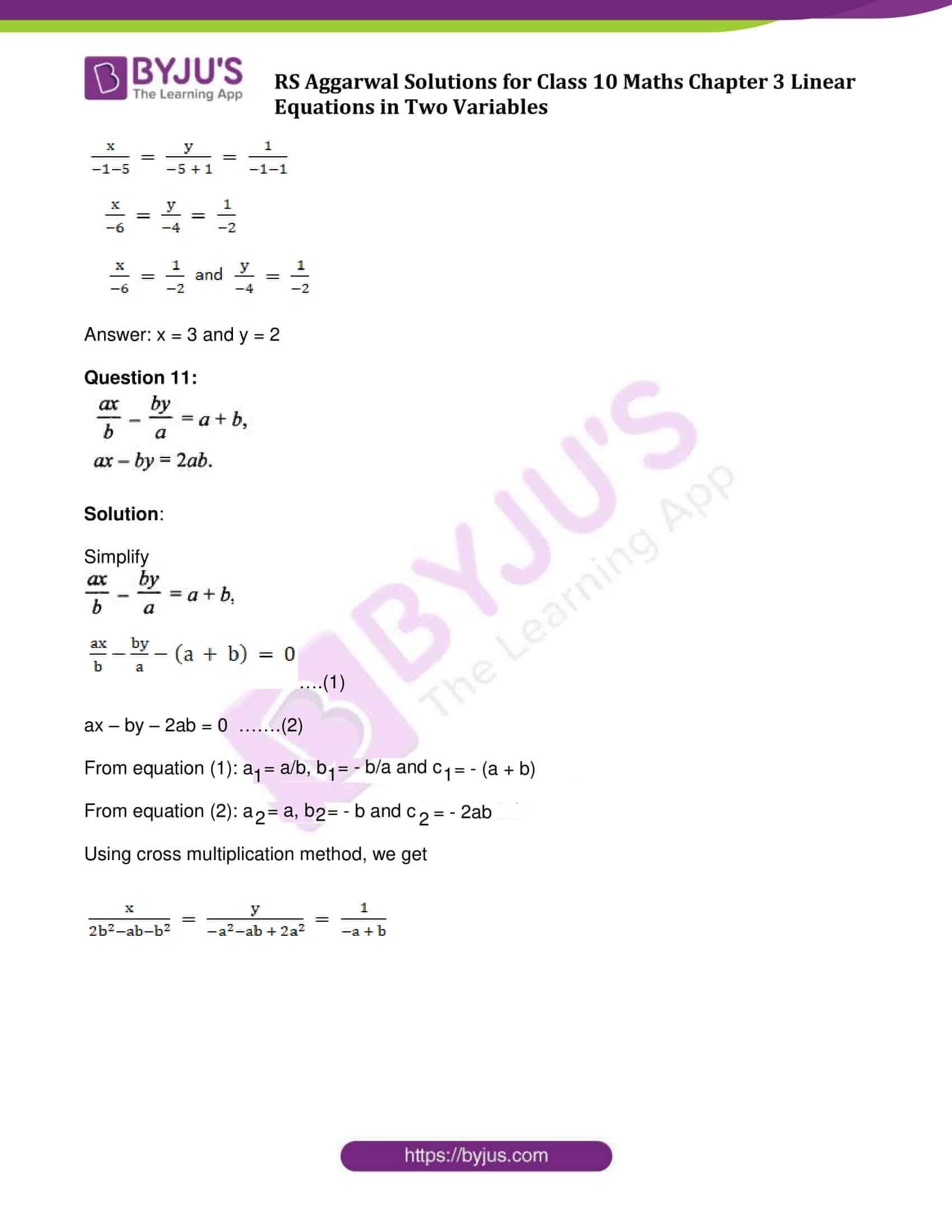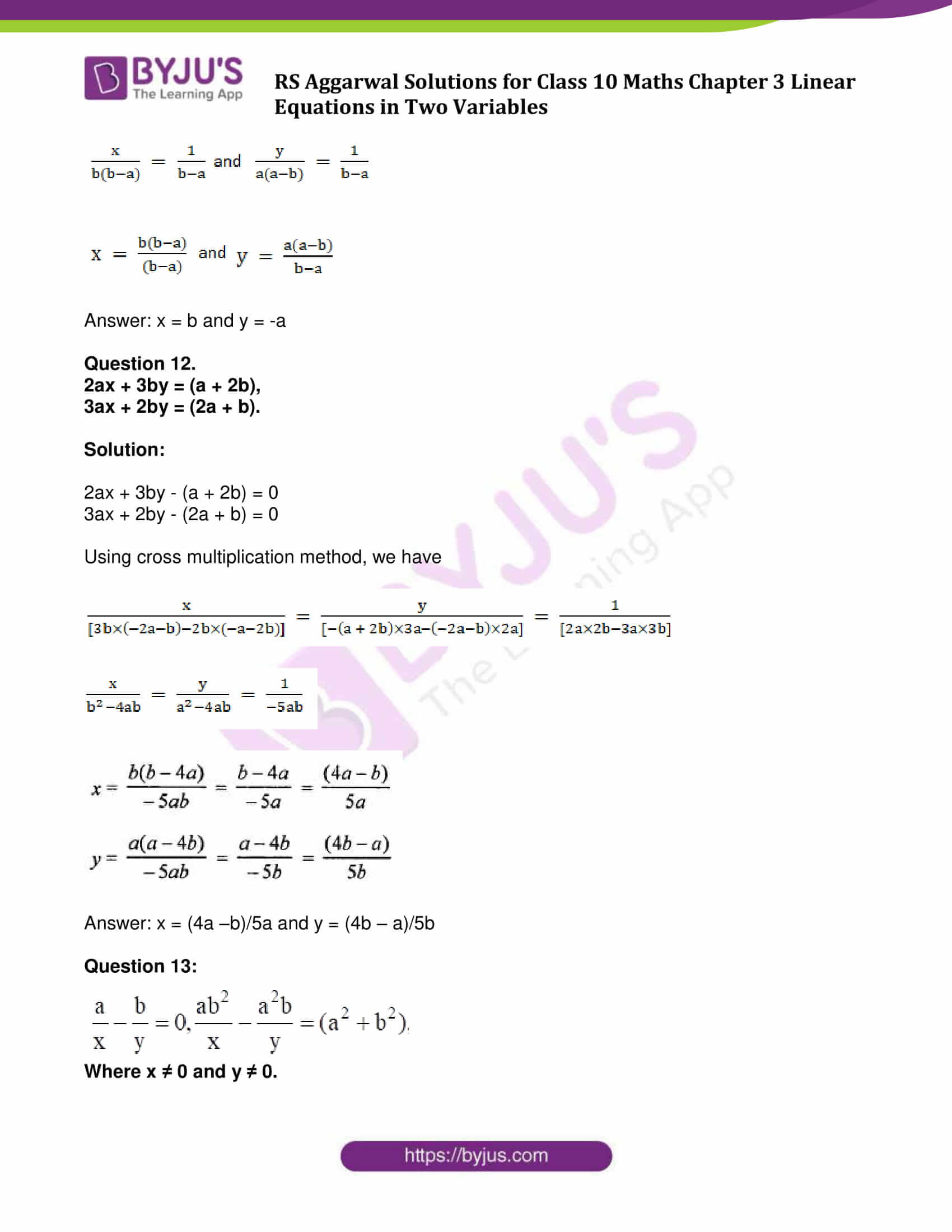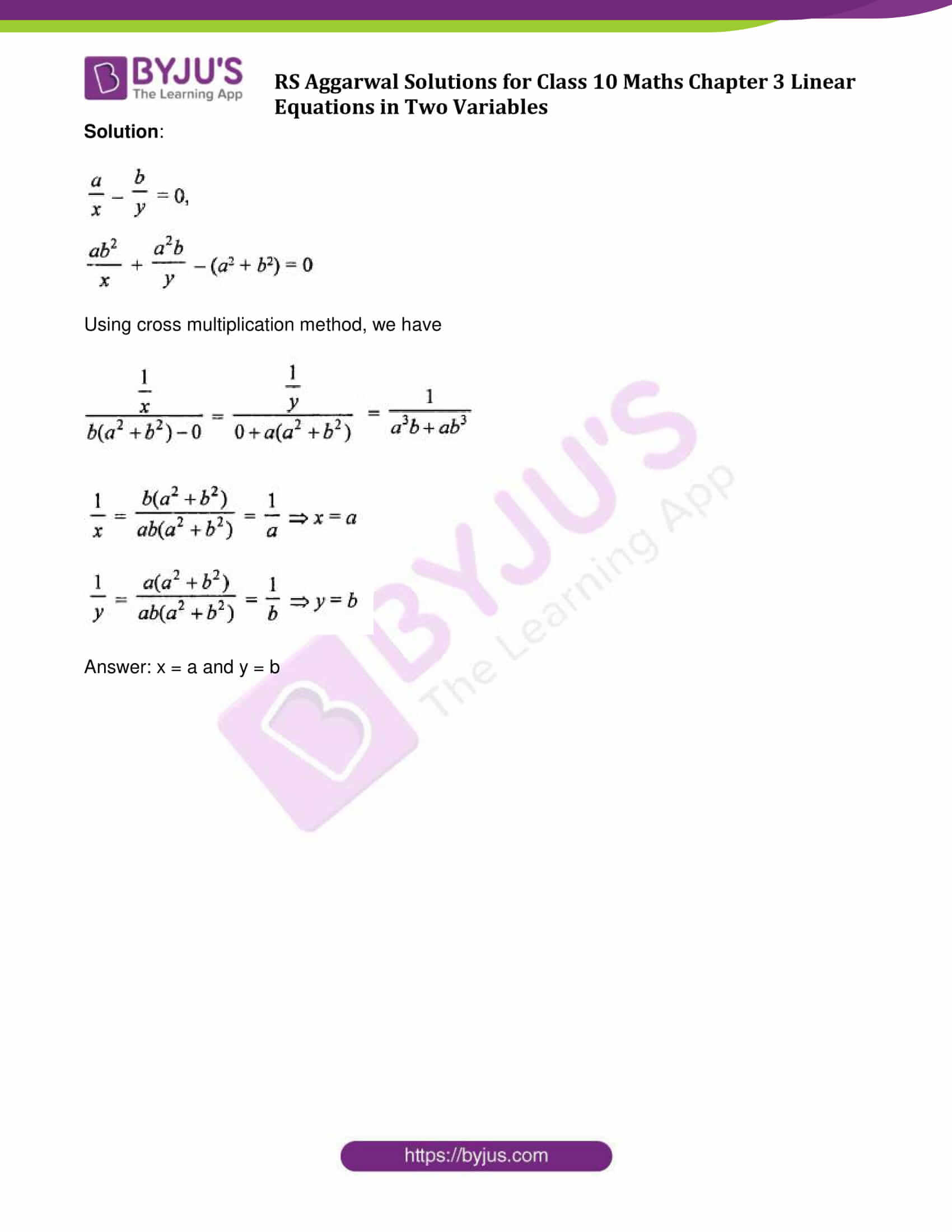### Access other exercise solutions of Class 10 Maths Chapter 3 Linear Equations in Two Variables

Exercise 3A Solutions: 29 Questions (Long Answers)

Exercise 3B Solutions: 20 Questions (Short Answers)

Exercise 3D Solutions: 14 Questions (Short Answers)

Exercise 3E Solutions: 20 Questions (Long Answers)

Exercise 3F Solutions: 10 Questions (6 Short Answers and 4 Long Answers)

## Exercise 3C Page No: 111

Solve each of the following systems of equations by using the method of cross multiplication:

Question 1:

x + 2y + 1 = 0,

2x – 3y – 12 = 0.

Solution:

x + 2y + 1 = 0 …(1)

2x – 3y – 12 = 0 …(2)

From equation (1): a1 = 1, b1 = 2 and c1 = 1

And from equation (2): a2 = 2, b2 = – 3 and c2 = – 12

Using cross multiplication method: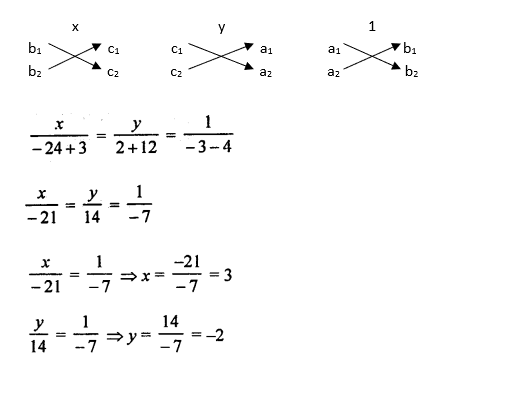Answer: x = 3 and y = – 2

Question 2:

3x – 2y + 3 = 0,

4x + 3y – 47 = 0.

Solution:

3x – 2y + 3 = 0 …(1)

4x + 3y – 47 = 0 …(2)

From equation (1): a1 = 3, b1 = – 2 and c1 = 3

From equation (2): a2 = 4, b2 = 3 and c2 = – 47

Using cross multiplication method: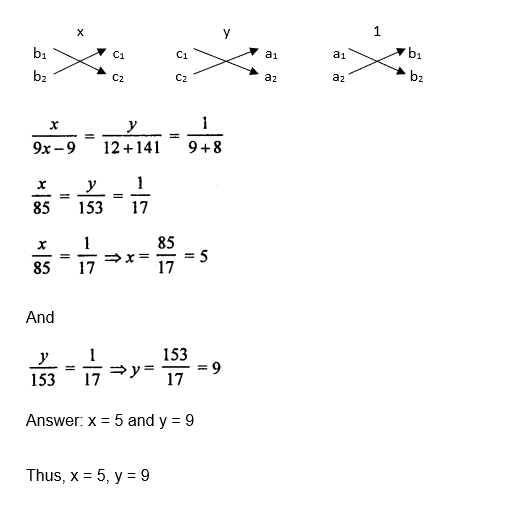Question 3:

6x – 5y – 16 = 0,

7x – 13y + 10 = 0.

Solution:

6x – 5y – 16 = 0 …(1)

7x – 13y + 10 = 0 …(2)

From equation (1): a1 = 6, b1 = – 5 and c1 = – 16

From equation (2): a2 = 7, b2 = – 13 and c2 = 10

Using cross multiplication method: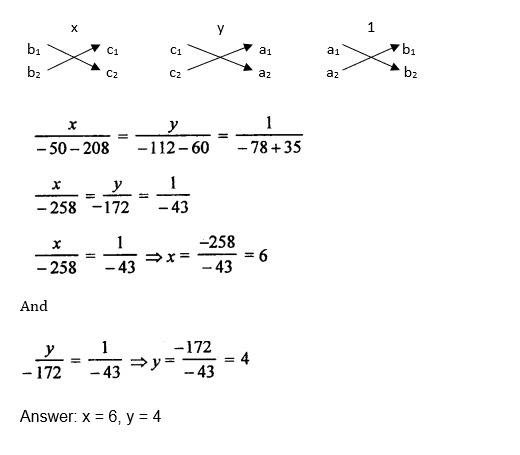Question 4:

3x + 2y + 25 = 0,

2x + y + 10 = 0.

Solution:

3x + 2y + 25 = 0 …(1)

2x + y + 10 = 0 …(2)

From equation (1): a1 = 3, b1 = 2 and c1 = 25

From equation (2): a2 = 2, b2 = 1 and c2 = 10

Using cross multiplication method: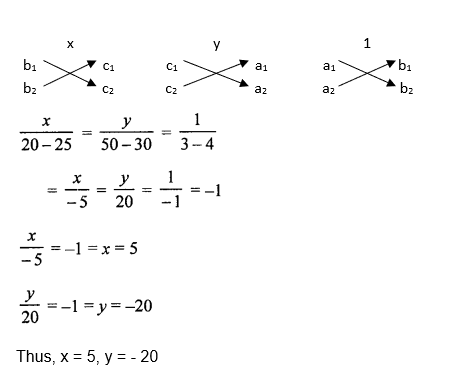Question 5:

2x + 5y = 1,

2x + 3y = 3.

Solution:

2x + 5y – 1 = 0 …(1)

2x + 3y – 3 = 0 …(2)

From equation (1): a1 = 2, b1 = 5 and c1 = – 1

From equation (2): a2 = 2, b2 = 3 and c2 = – 3

Using cross multiplication method: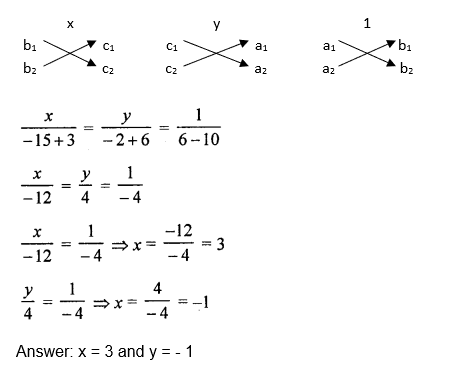Question 6:

2x + y = 35,

3x + 4y = 65.

Solution:

2x + y – 35 = 0 …(1)

3x + 4y – 65 = 0 …(2)

From equation (1): a1 = 2, b1 = 1 and c1 = – 35

From equation (2): a2 = 3, b2 = 4 and c2 = – 65

Using cross multiplication method: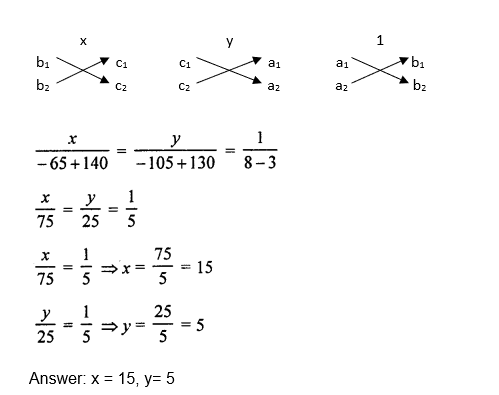Question 7:

7x – 2y = 3,

22x – 3y = 16.

Solution:

7x – 2y – 3 = 0 …(1)

22x – 3y – 16 = 0 …(2)

From equation (1): a1 = 7, b1 = – 2 and c1 = – 3

From equation (2): a2 = 22, b2 = – 3 and c2 = – 16

Using cross multiplication method: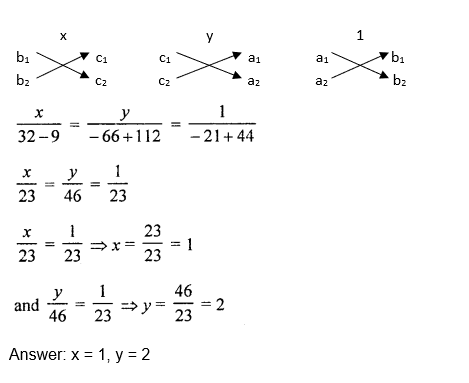Question 8.

x/6 + y/15 = 4

x/3 – y/12 = 19/4

Solution:

Simplify given equations:

x/6 + y/15 = 4

(5x + 2y)/30 = 4

5x + 2y – 120 = 0

And x/3 – y/12 = 19/4

(4x – y)/12 = 19/4

4x – y – 57 = 0

New set of equations is:

5x + 2y – 120 = 0 …..(1)

4x – y – 57 = 0 ……….(2)

From equation (1): a1 = 5, b1 = 2 and c1 = – 120

From equation (2): a2 = 4, b2 = – 1 and c2 = – 57

Using cross multiplication,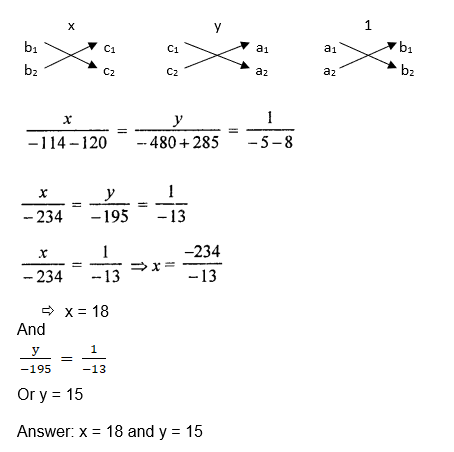Question 9:

1/x + 1/y = 7

2/x + 3/y = 17 (x≠0 and y≠ 0)

Solution:

Simplify given equations:

Let 1/x = u and 1/y = v

1/x + 1/y = 7 implies

u + v = 7

2/x + 3/y = 17 implies:

2u + 3v = 17

New set of equations is:

u + v = 7 ……(1)

2u + 3v = 17 ………(2)

From equation (1): a1 = 1, b1 = 1 and c1 = – 7

From equation (2): a2 = 2, b2 = 3 and c2 = – 17

Using cross multiplication method: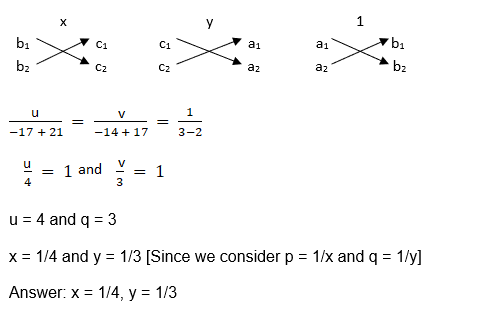Question 10: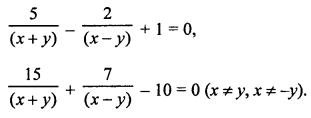Solution:

Consider 1/(x + y) = a and 1/(x – y) = b

Given equations turn as:

5a – 2b + 1 = 0 ….(1)

15a + 7b – 10 = 0 …(2)

From equation (1): a1 = 5, b1 = -2 and c1 = 1

From equation (2): a2 = 15, b2 = 7 and c2 = – 10

Using cross multiplication method: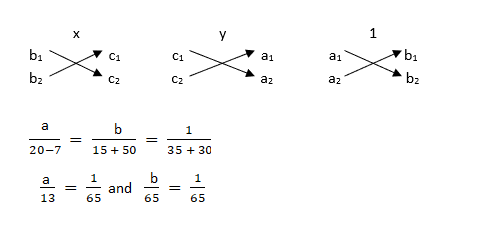So a = 13/65 = 1/5 and b = 65/65 = 1

Putting back values and write equations in the form of x and y, we get

x + y = 5 and x – y = 1

Again we get a set of two linear equations with two variables. Solve again to find the value of x and y.

Rearranging them again,

x + y – 5 = 0 …(3)

x – y – 1 = 0 …(4)

From equation (3): a1 = 1, b1 = 1 and c1 = – 5

From equation (4): a2 = 1, b2 = – 1 and c2 = – 1

Using cross multiplication method,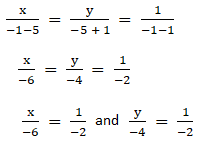Answer: x = 3 and y = 2

Question 11: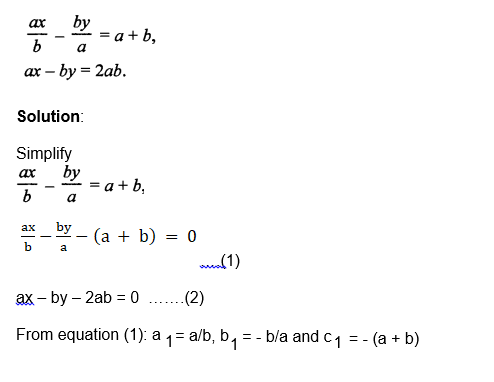From equation (2): a2 = a, b2 = – b and c2 = – 2ab

Using cross multiplication method, we get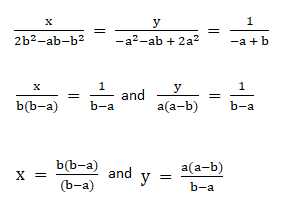Answer: x = b and y = -a

Question 12.

2ax + 3by = (a + 2b),

3ax + 2by = (2a + b).

Solution: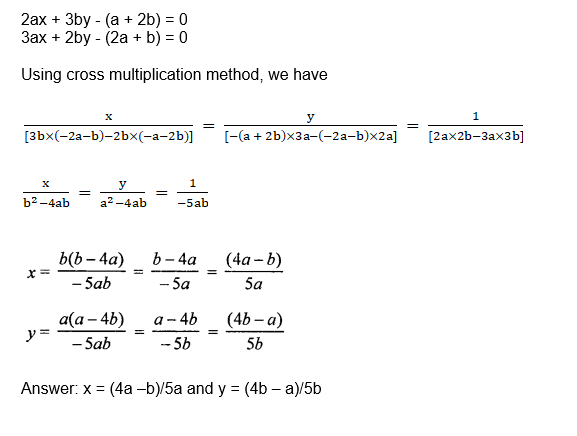Question 13: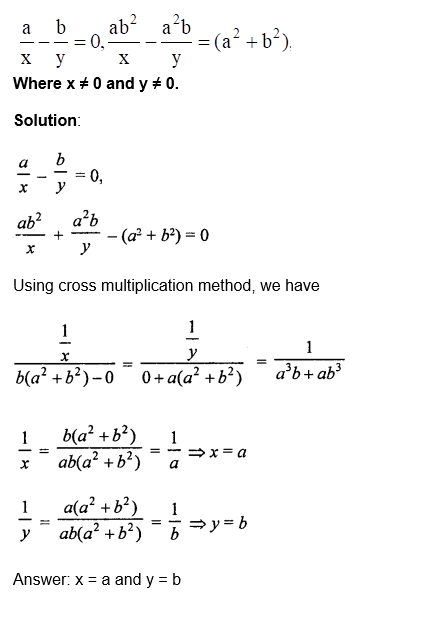## RS Aggarwal Solutions for Class 10 Maths Chapter 3 Linear Equations in Two Variables Exercise 3C

Class 10 Maths Chapter 3 Linear Equations in Two Variables Exercise 3C is based on the topic: Solving simultaneous linear equations using the method of cross multiplication.